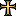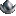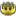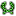# Few Muslim events.

#### unmerged(105862)

##### Banned
••••Some numbers can be bit exaggerated so i don't mind your critique:

Code:
``````##############################
# Some muslim related events #
##############################

# Some people don't like pork
province_event = {
id = 50000 # id numeration is random - i numbered them as i went along no actual connection with the event type or context ...

picture = "event_muslim"

trigger = {
condition = { type = or
condition = { type = religion value = moslem }
condition = { type = religion value = jewish }
}
condition = { type = or
condition = { type = ruler_religion value = moslem }
condition = { type = ruler_religion value = jewish }
}
condition = { type = has_advance value = { pigs = yes } }
}

mean_time_to_happen = {
months = 12

modifier = {
condition = { type = trait value = zealous }
factor = 0.5
}
modifier = {
condition = { type = trait value = arbitrary }
factor = 0.5
}
modifier = {
condition = { type = trait value = cruel }
factor = 0.5
}
modifier = {
condition = { type = trait value = detached_priest }
factor = 0.5
}
modifier = {
condition = { type = trait value = maniac }
factor = 0.5
}
modifier = {
condition = { type = trait value = pragmatic }
factor = 0.5
}
modifier = {
condition = { type = trait value = proud }
factor = 0.5
}
modifier = {
condition = { type = trait value = reckless }
factor = 0.5
}
}

immidiate = {
effect = { type = remove_advance value = pigs  }
effect = { type = prosperity value = -1 }
effect = { type = noble_loyalty value = 0.25 }
effect = { type = clergy_loyalty value = 0.25 }
effect = { type = burgher_loyalty value = 0.25 }
effect = { type = peasant_loyalty value = 0.25 }
}
}

# Some people like pork, some don't
province_event = {
id = 50001

picture = "event_muslim"

trigger = {
condition = { type = ruler_religion value = moslem } # Jewish rulers aren't that oppressive, they can actually live side-by-side
condition = { type = has_advance value = { pigs = yes } }
condition = { type = not value = { type = religion value = moslem } }
condition = { type = not value = { type = religion value = jewish } } # They don't mind either
}

mean_time_to_happen = {
months = 12

modifier = {
condition = { type = trait value = zealous }
factor = 0.5
}
modifier = {
condition = { type = trait value = arbitrary }
factor = 0.5
}
modifier = {
condition = { type = trait value = cruel }
factor = 0.5
}
modifier = {
condition = { type = trait value = detached_priest }
factor = 0.5
}
modifier = {
condition = { type = trait value = maniac }
factor = 0.5
}
modifier = {
condition = { type = trait value = pragmatic }
factor = 0.5
}
modifier = {
condition = { type = trait value = proud }
factor = 0.5
}
modifier = {
condition = { type = trait value = reckless }
factor = 0.5
}
}

immidiate = {
effect = { type = remove_advance value = pigs  }
effect = { type = prosperity value = -1 }
effect = { type = add_province_effect value = revolt } # Wtf man
effect = { type = add_province_effect value = looted }
effect = { type = noble_loyalty value = -0.75 } # Wtf, we want to eat something
effect = { type = clergy_loyalty value = -0.75 }
effect = { type = burgher_loyalty value = -0.75 }
effect = { type = peasant_loyalty value = -0.75 }
}
}

# Some people just don't like beer
province_event = {
id = 50002

picture = "event_muslim"

trigger = {
condition = { type = ruler_religion value = moslem }
condition = { type = has_improvement value = { brewery = yes } }
condition = { type = religion value = moslem }
}

mean_time_to_happen = {
months = 12

modifier = {
condition = { type = trait value = zealous }
factor = 0.5
}
modifier = {
condition = { type = trait value = arbitrary }
factor = 0.5
}
modifier = {
condition = { type = trait value = cruel }
factor = 0.5
}
modifier = {
condition = { type = trait value = detached_priest }
factor = 0.5
}
modifier = {
condition = { type = trait value = maniac }
factor = 0.5
}
modifier = {
condition = { type = trait value = pragmatic }
factor = 0.5
}
modifier = {
condition = { type = trait value = proud }
factor = 0.5
}
modifier = {
condition = { type = trait value = reckless }
factor = 0.5
}
}

immidiate = {
effect = { type = remove_improvement value = brewery  }
effect = { type = prosperity value = -1 }
effect = { type = noble_loyalty value = 0.25 } # Allah-Uakhbarr !!!
effect = { type = clergy_loyalty value = 0.25 }
effect = { type = burgher_loyalty value = 0.25 }
effect = { type = peasant_loyalty value = 0.25 }
}
}

# Some people like beer - some don't
province_event = {
id = 50003

picture = "event_muslim"

trigger = {
condition = { type = ruler_religion value = moslem }
condition = { type = has_improvement value = { brewery = yes } }
condition = { type = not value = { type = religion value = moslem } }
}

mean_time_to_happen = {
months = 12

modifier = {
condition = { type = trait value = zealous }
factor = 0.5
}
modifier = {
condition = { type = trait value = arbitrary }
factor = 0.5
}
modifier = {
condition = { type = trait value = cruel }
factor = 0.5
}
modifier = {
condition = { type = trait value = detached_priest }
factor = 0.5
}
modifier = {
condition = { type = trait value = maniac }
factor = 0.5
}
modifier = {
condition = { type = trait value = pragmatic }
factor = 0.5
}
modifier = {
condition = { type = trait value = proud }
factor = 0.5
}
modifier = {
condition = { type = trait value = reckless }
factor = 0.5
}
}

immidiate = {
effect = { type = remove_improvement value = brewery  }
effect = { type = prosperity value = -2 }
effect = { type = add_improvement value = thieves_guild } # Those who can't brew will take it from others
effect = { type = add_improvement value = smugglers_ring } # We'll brew some ourselves and distribute it to people
effect = { type = add_improvement value = highway_robber_band } # We want some compensation
effect = { type = add_province_effect value = revolt } # Wtf - we will express our displeasment
effect = { type = add_province_effect value = looted } # We'll riot a bit
effect = { type = noble_loyalty value = -1.0 } # Wtf = we don't like this a bit
effect = { type = clergy_loyalty value = -1.0 } # We miss our holy communion
effect = { type = burgher_loyalty value = -1.0 } # Wtf - how can we relax after hard day's night
effect = { type = peasant_loyalty value = -1.0 } # Wtf - you must be kidding
}
}``````

In my games muslims always tended to get the upper hand, so i thought that history and religious tradition would help me out. What do you think ?